2021-10-03

Recap

Prev: Reproducibility

• 221 Reproducibility
• 222 R and Markdown
• 223 Git
• 224 Practical session

Now: Data visualisation

• Grammar of graphics
• ggplot2

Grammar of graphics

Grammars provide rules for languages

“The grammar of graphics takes us beyond a limited set of charts (words) to an almost unlimited world of graphical forms (statements)” (Wilkinson, 2005)

Statistical graphic specifications are expressed in six statements:

1. Data manipulation
2. Variable transformations (e.g., rank),
3. Scale transformations (e.g., log),
4. Coordinate system transformations (e.g., polar),
5. Element: mark (e.g., points) and visual variables (e.g., color)
6. Guides (axes, legends, etc.).

Visual variables

A visual variable is an aspect of a mark that can be controlled to change its appearance.

Visual variables include:

• Size
• Shape
• Orientation
• Colour (hue)
• Colour value (brightness)
• Texture
• Position (2 dimensions)

ggplot2

The ggplot2 library offers a series of functions for creating graphics declaratively, based on the Grammar of Graphics.

To create a graph in ggplot2:

• provide the data
• specify elements
• which visual variables (aes)
• which marks (e.g., geom_point)
• apply transformations
• guides

Aesthetics

The aes element provides a “mapping” from the data columns (attributes) to the graphic’s visual variables, including:

• x and y
• fill (fill colour) and colour (border colour)
• shape
• size
data %>%
ggplot2::ggplot(
aes(
x = column_1,
y = column_2
)
)

Graphical primitives

Marks (graphical primitives) can be specified through a series of functions, such as geom_line, geom_bar or geom_point

These can be added to the construction of the graph using +

ggplot2::ggplot(
aes(
x = column_1, y = column_2
)
) +
ggplot2::geom_line()

ggplot2::geom_line

• x: a column to “map” to the x-axis, e.g. days (category)
• y: a column to “map” to the y-axis, e.g. delay (continuous)
• ggplot2::geom_line: line mark (graphical primitive)
nycflights13::flights %>%
dplyr::filter(!is.na(dep_delay) & month == 11) %>%
dplyr::mutate(flight_date = ISOdate(year, month, day)) %>%
dplyr::group_by(flight_date) %>%
dplyr::summarize(tot_dep_delay = sum(dep_delay)) %>%
ggplot2::ggplot(aes(
x = flight_date,
y = tot_dep_delay
)) +
ggplot2::geom_line()

ggplot2::geom_line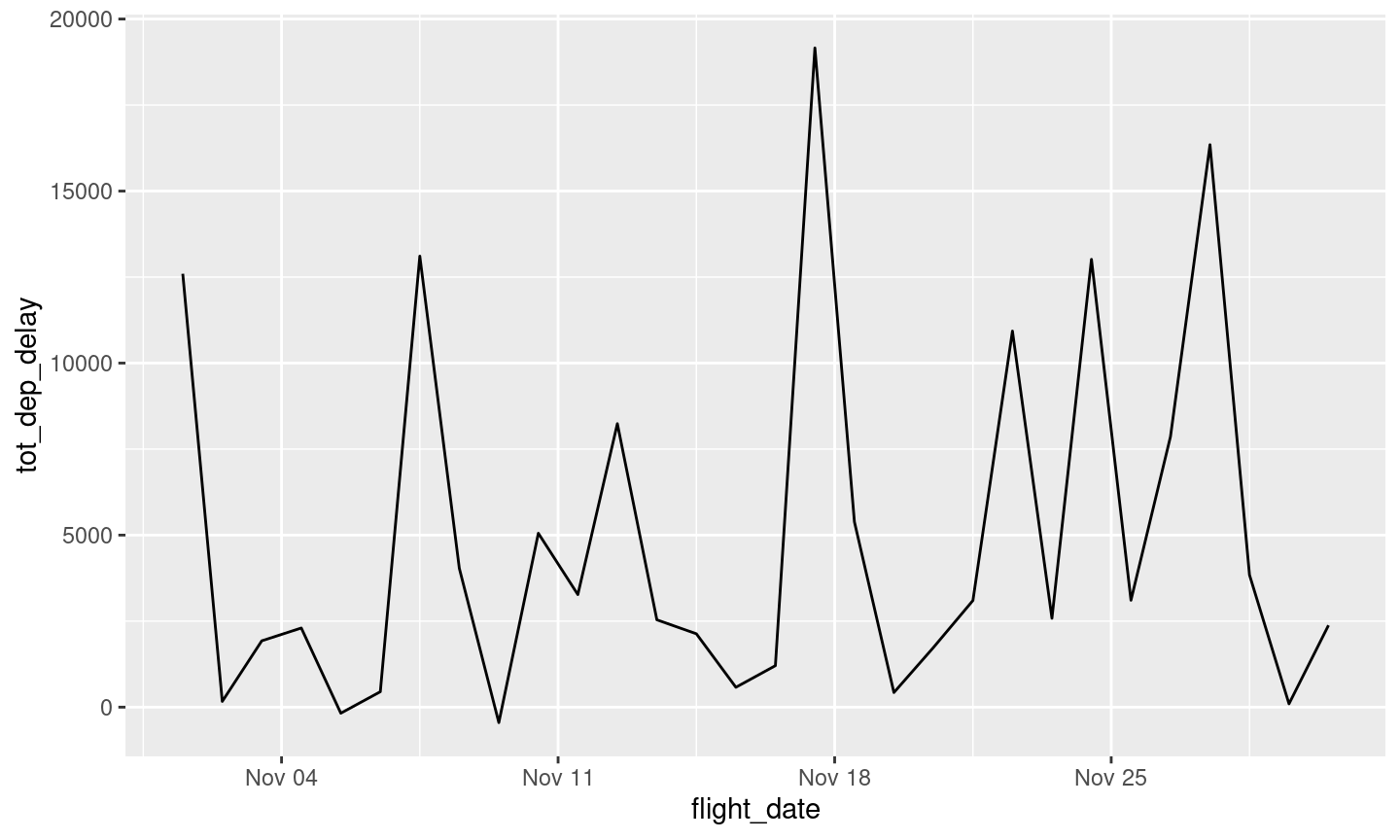ggplot2::geom_col

• x: a column to “map” to the x-axis, e.g. days (category)
• y: a column to “map” to the y-axis, e.g. delay (continuous)
• ggplot2::geom_col: bar mark (graphical primitive)
• ggplot2::geom_bar instead illustrates count per category
nycflights13::flights %>%
dplyr::filter(!is.na(dep_delay) & month == 11) %>%
dplyr::mutate(flight_date = ISOdate(year, month, day)) %>%
dplyr::group_by(flight_date) %>%
dplyr::summarize(tot_dep_delay = sum(dep_delay)) %>%
ggplot2::ggplot(aes(
x = flight_date,
y = tot_dep_delay
)) +
ggplot2::geom_col()

ggplot2::geom_col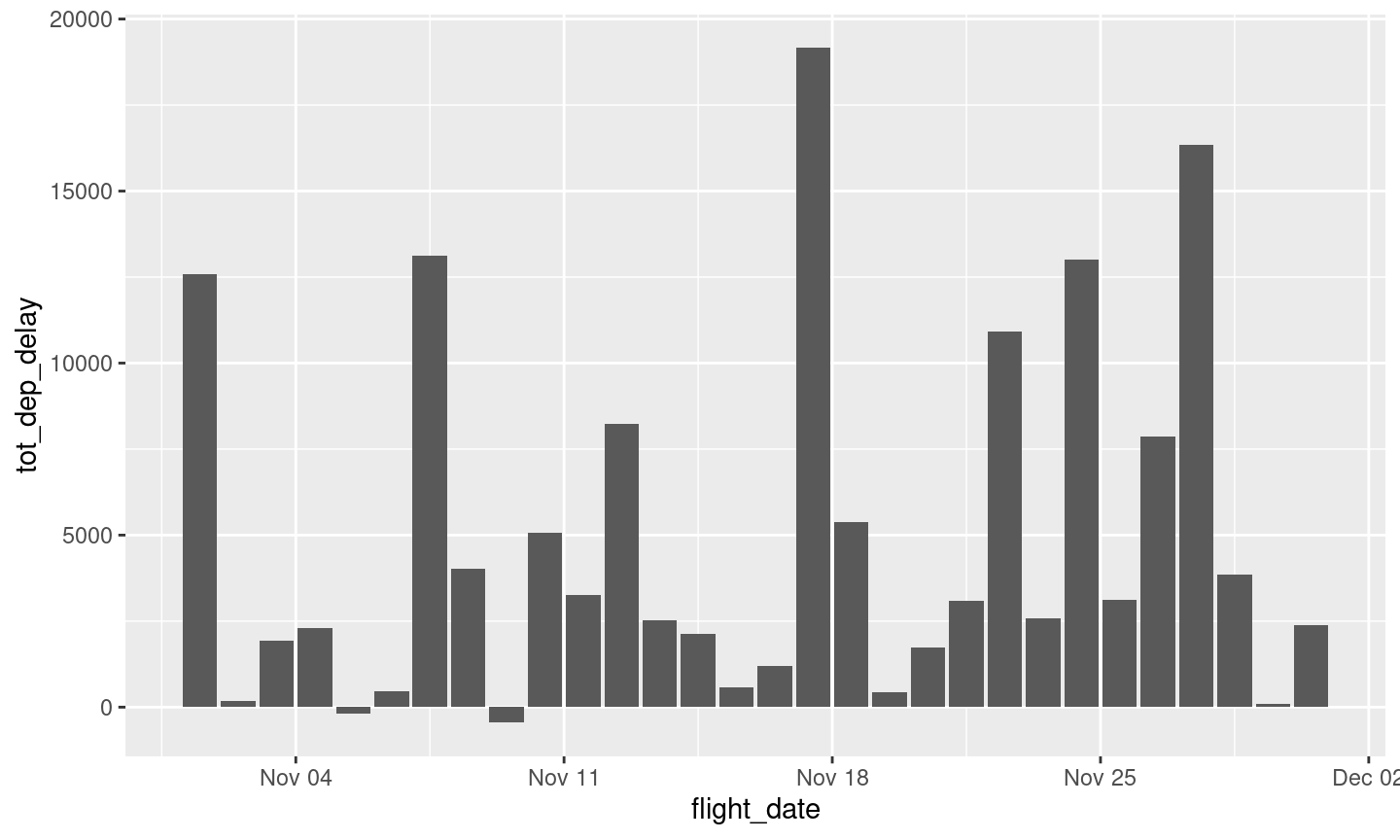ggplot2::geom_col

… then, why not add some colour?

• fill: a column to “map” to the visual variable colour as fill of the mark, e.g. origin (category)
• colour can be used to “map” a column to the visual variable colour as border of the mark
nycflights13::flights %>%
dplyr::filter(!is.na(dep_delay) & month == 11) %>%
dplyr::mutate(flight_date = ISOdate(year, month, day)) %>%
dplyr::group_by(flight_date, origin) %>%
dplyr::summarize(tot_dep_delay = sum(dep_delay)) %>%
ggplot2::ggplot(aes(
x = flight_date,
y = tot_dep_delay,
fill = origin
)) +
ggplot2::geom_col()

ggplot2::geom_col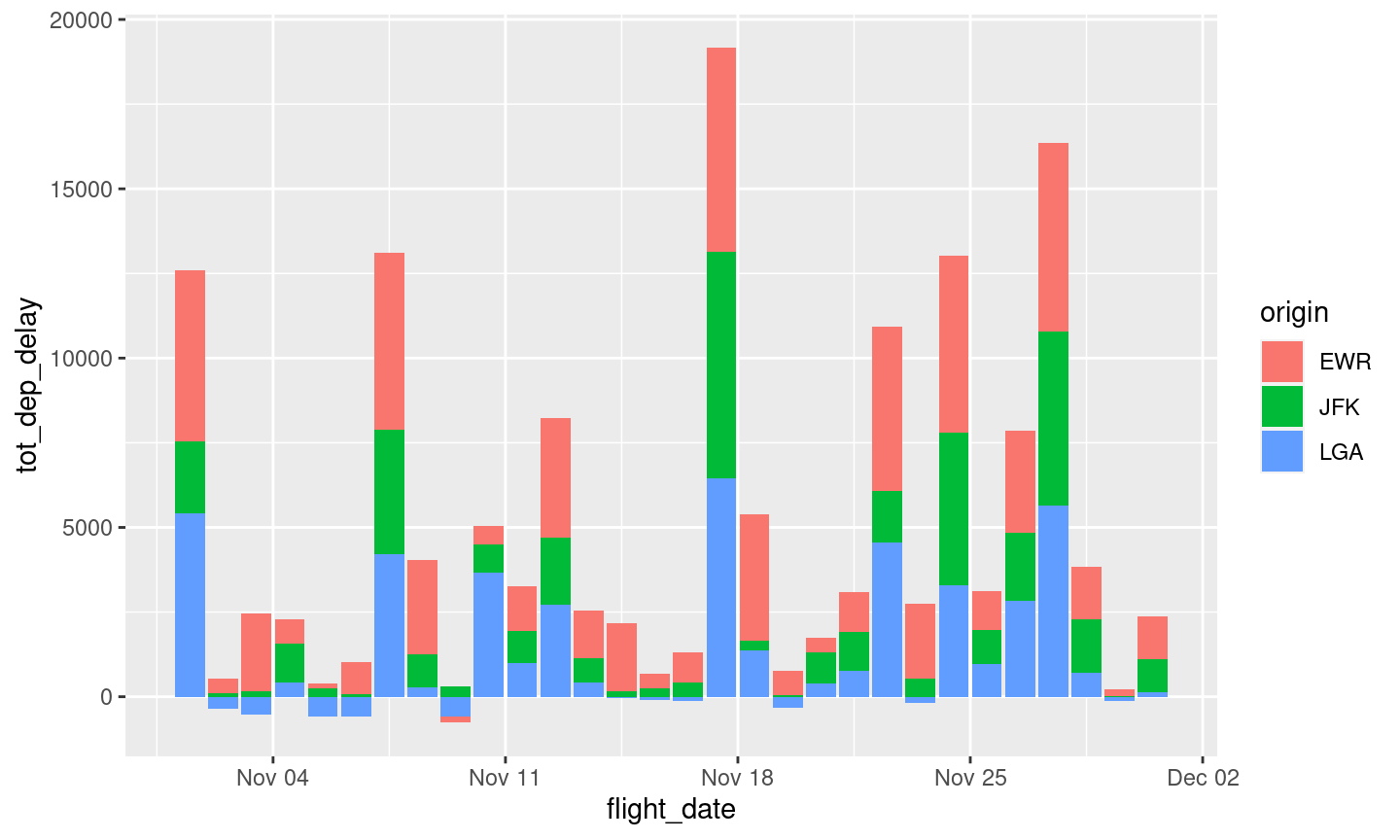Histograms

• x a column to “map” to the x-axis, e.g. delay (continuous)
• ggplot2::geom_histogram to illustrate count over intervals of continuous variable on x-axis
• ggplot2::geom_bar instead illustrates count per category
nycflights13::flights %>%
dplyr::filter(month == 11) %>%
ggplot2::ggplot(
aes(
x = dep_delay
)
) +
ggplot2::geom_histogram(
binwidth = 10
)

Histograms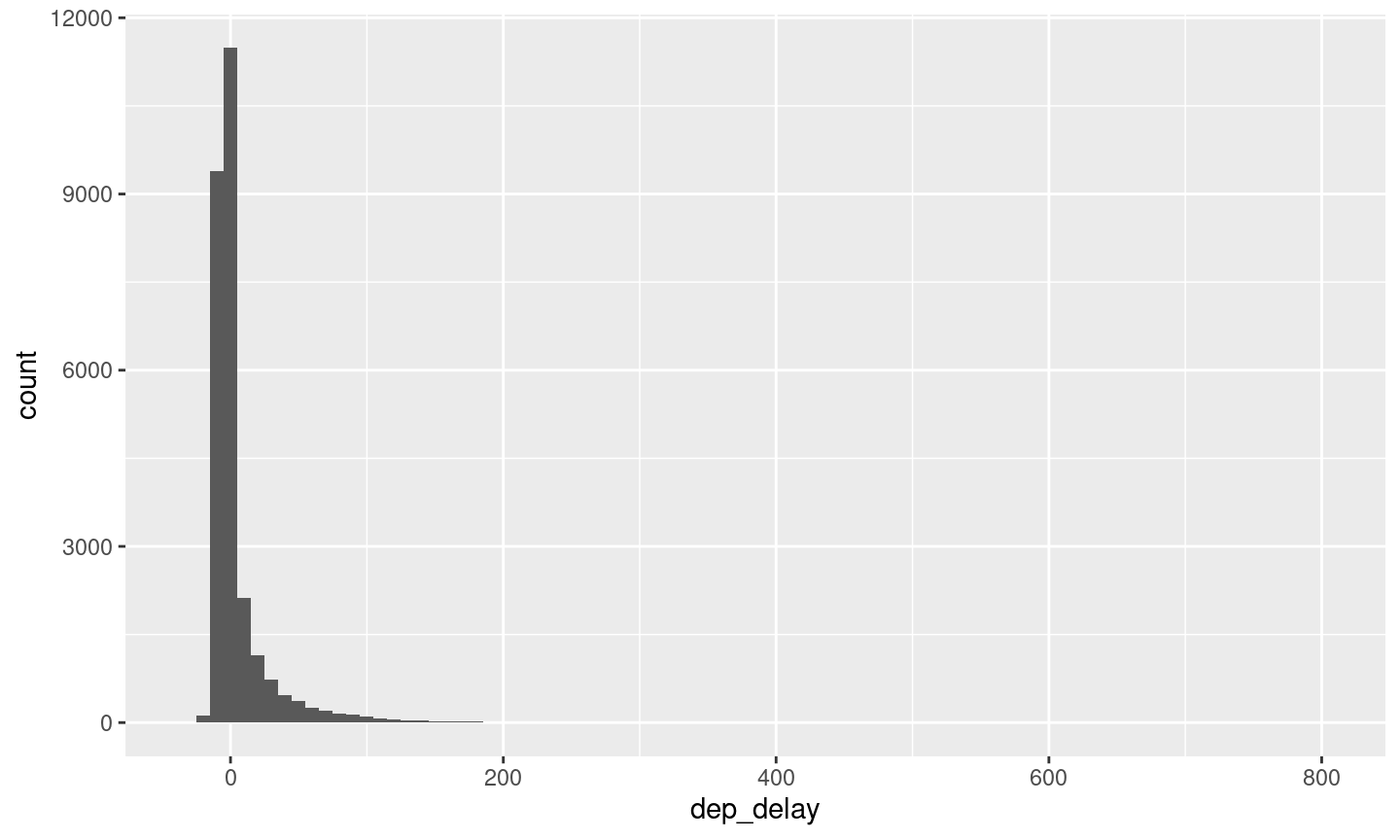Scatterplots

• x and y variables to plot
• ggplot2::geom_point
nycflights13::flights %>%
dplyr::filter(
month == 11,
carrier == "US",
!is.na(dep_delay),
!is.na(arr_delay)
) %>%
ggplot2::ggplot(aes(
x = dep_delay,
y = arr_delay
)) +
ggplot2::geom_point()

Scatterplots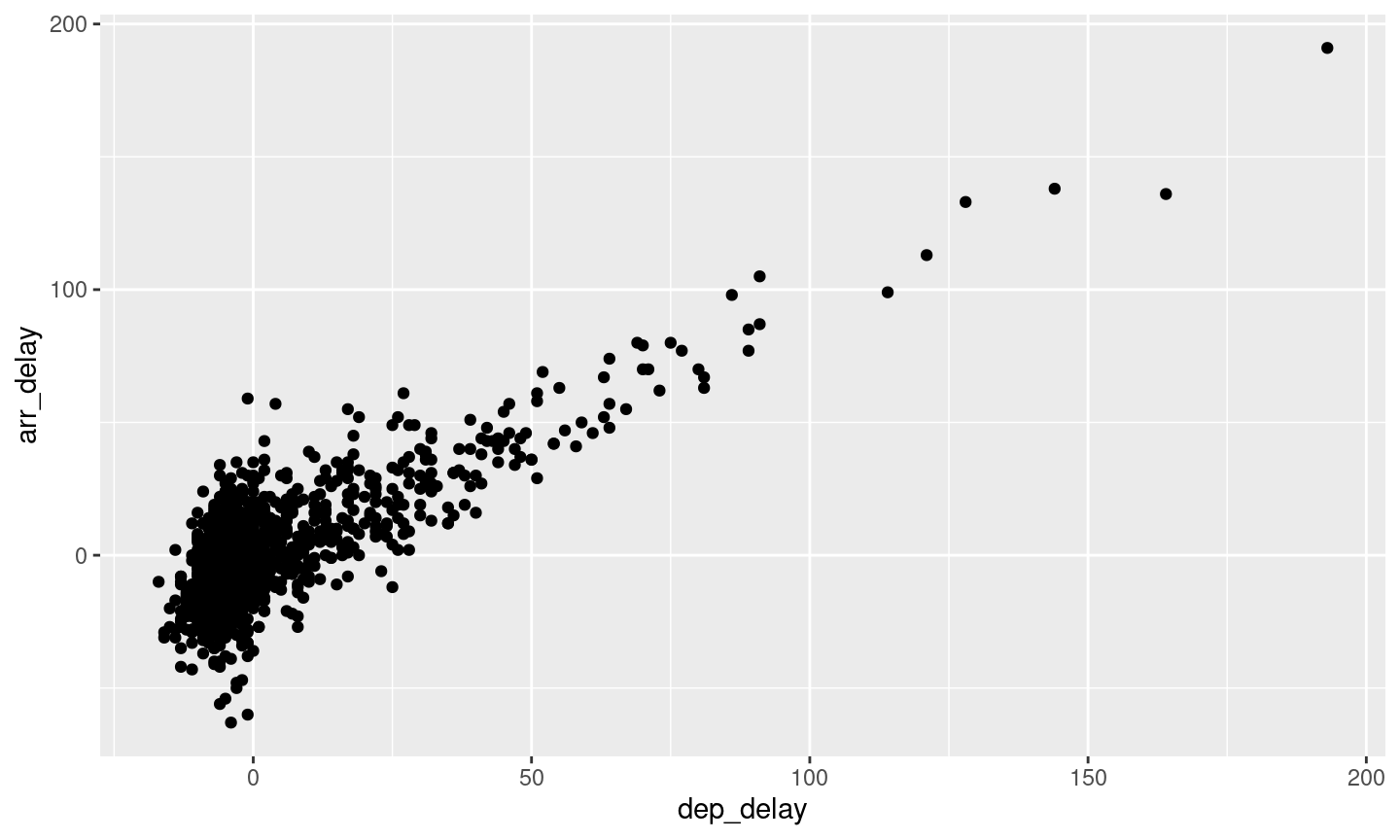Overlapping points

• x and y variables to plot
• ggplot2::geom_count counts overlapping points and maps the count to size
nycflights13::flights %>%
dplyr::filter(
month == 11, carrier == "US",
!is.na(dep_delay), !is.na(arr_delay)
) %>%
ggplot2::ggplot(aes(
x = dep_delay,
y = arr_delay
)) +
ggplot2::geom_count()

Overlapping points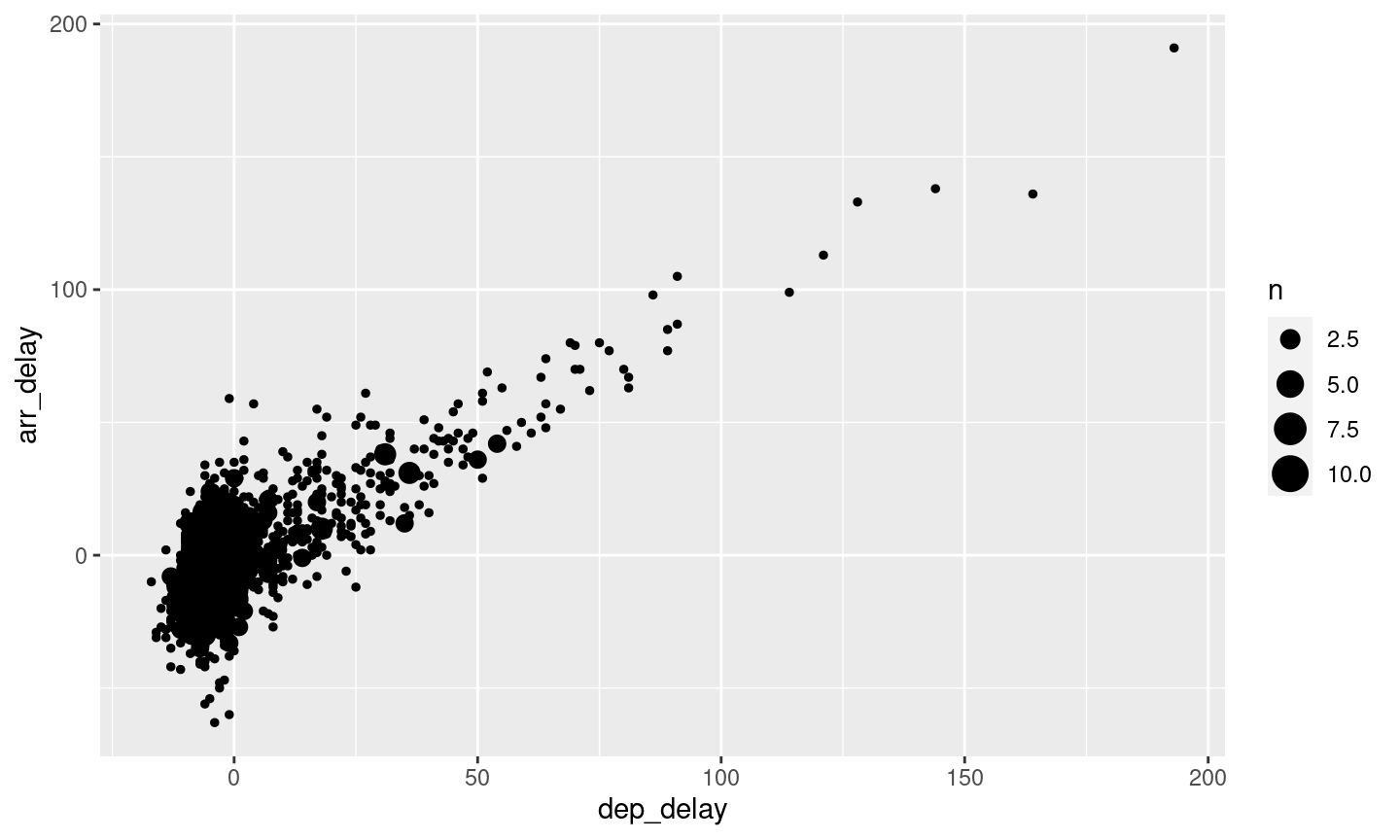Bin counts

• x and y variables to plot
• ggplot2::geom_bin2d with 10 minutes binwidth
nycflights13::flights %>%
dplyr::filter(
month == 11,
carrier == "US",
!is.na(dep_delay),
!is.na(arr_delay)
) %>%
ggplot2::ggplot(aes(
x = dep_delay,
y = arr_delay
)) +
ggplot2::geom_bin2d(binwidth = 10)

Bin counts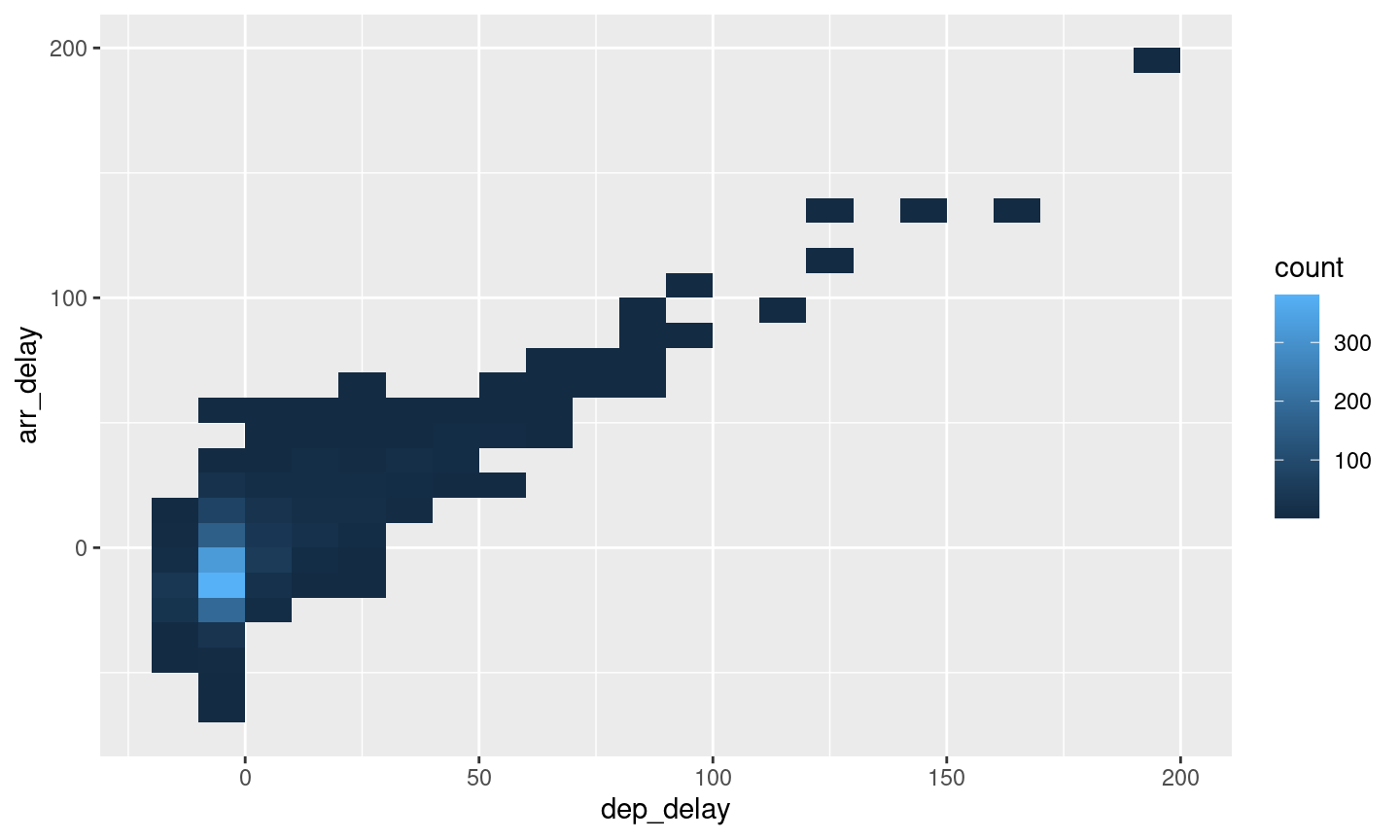Coordinates transformations

• ggplot2::coord_fixed manipulates coordinates property
• ggplot2::theme_bw classic dark-on-light theme
nycflights13::flights %>%
dplyr::filter(
month == 11,
carrier == "US",
!is.na(dep_delay),
!is.na(arr_delay)
) %>%
ggplot2::ggplot(aes(
x = dep_delay,
y = arr_delay
)) +
ggplot2::geom_bin2d(binwidth = 10) +
ggplot2::coord_fixed(ratio = 1) +
theme_bw()

Coordinates transformations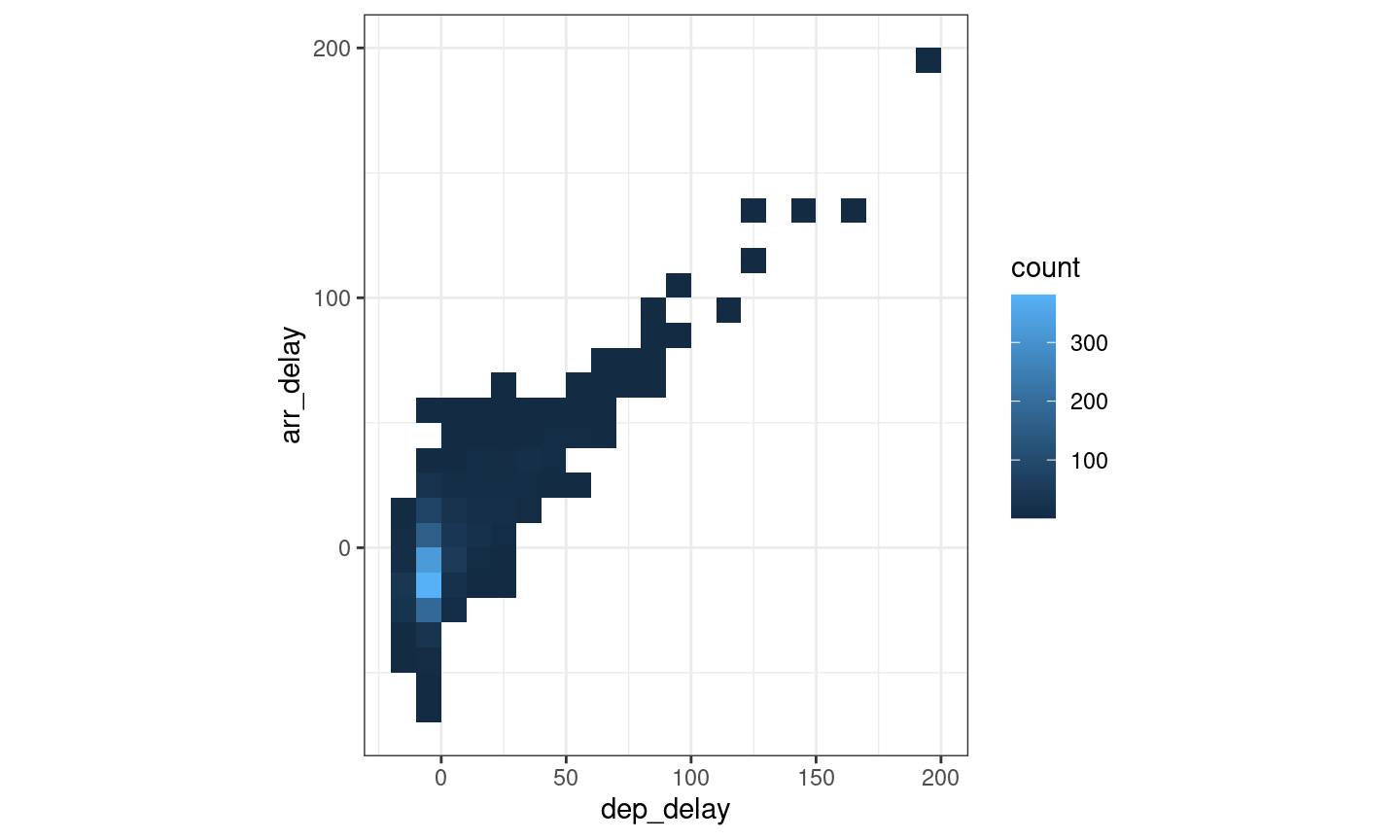Summary

Data visualisation

• Grammar of graphics
• ggplot2

Next: Descriptive statistics

• pastecs::stat.desc
• dplyr::across